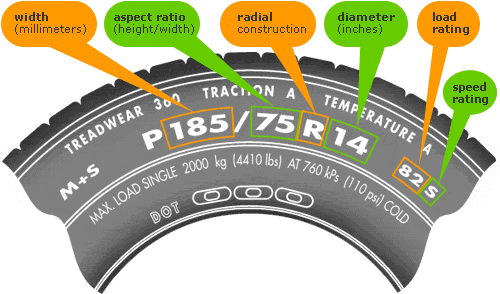## You can change this text in the options panel in the admin

There are tons of ways to configure Magazine Premium... The possibilities are endless!

# Calculating rim and tire sizes to achieve stock wheel diameter

January 24, 2012
By

So your thinking, man I really dont like those bottle caps (stock rims) on my E30. A mistake a lot of people make is they intuitively think that a bigger rim automatically equals a bigger overall wheel diameter. Tire sizing is determined by calculating the mm width of the tread, percentage of that width for the height of the sidewall, and the rim size it fits:In the above picture the tread will be 185mm wide, the side wall height will be 75% of the height of the the width (185 x .75 = 138.75mm), and it will fit a 14 inch rim.

A stock rim and tire combo on a non-M e30 is 195/65/14 again that means the tread is 195mm wide, the sidewall height is 65% of the width (195 x .65 = 126.75) fitted on a 14 inch rim.

Now to understand the overall diameter of the rim and tire, we calculate ((195*.65)/25.4*2)+14 = 23.980315 inches

So 23.980315 inches is the stock diameter of a wheel on a non-M E30, that is what the speedometer is calibrated to, and that is the height the car will be at the height it was designed to be at (center of gravity). The way we calculated that is by calculating the sidewall height of the tire (195*.65) we divide that by 25.4 to convert from mm to inches then multiply it by 2 because a wheel is round and you need to add the diameter of the rim plus each side of the rubber sidewall.

An E30 M3, and basket weave style rims from europe are 15 inch (irrelevant: but the m3 has a 5 lug setup). Intuitively you would think that it runs a bigger wheel.  Well lets test that theory: the M3 uses a 205/55/15 tire we see that actually it equals (((205 * .55) / 25.4) * 2) + 15 = 23.8779528 inches. Meaning that actually the overall diameter of the wheel on the M3 is ever so slightly smaller, 0.11 isnt much, in terms of tires that is pretty much the same, because tire ratios for the sidewall go in increments of 5 so 205/60/15  = 24.6850394 would be to big and 205/50/15 = 23.0708661 would be to small.

Also assume you get a rim that is the same 14 inch as your current stock rims, but these new rims are wider and you will be running wider tires. If you choose 215/65/14 you are not only changing the width but also the height and will end up with a (((215 * .65) / 25.4) * 2) + 14 = 25.003937 inch wheel. Preferably you need to get a 215/60/14 tire because it is the closest sidewall ratio to the original size (((215 * .60) / 25.4) * 2) + 14 = 24.1574803 inch.

For simplicity sake, say you have a non-M and want a 15 inch rim of the same width as your 14 inch rim you want a 195/60/15 tire  (((195 * .60) / 25.4) * 2) + 15 = 24.2125984.

The optimal sizes for:

16 inch: 195/50/16 (((195 * .50) / 25.4) * 2) + 16 = 23.6771654

17 inch: 195/45/17 (((195 * .45) / 25.4) * 2) + 17 = 23.9094488

18 inch: 195/40/18 (((195 * .40) / 25.4) * 2) + 18 = 24.1417323

Now just for fun say you have a 17 x 8 inch rim and you want the stock wheel diameter, the rim uses a 245mm wide tire, so you would want to run a 245/35/17 as (((245 * .35) / 25.4) * 2) + 17 = 23.7519685

I have embedded a calculator for your using pleasures: